# K means clustering

28/12/2019

## Concept

K means clustering is the most commonly used unsupervised machine learning algorithm for partitioning a given data set into a set of k groups. It classifies objects in multiple groups (i.e., clusters), such that objects within the same cluster are as similar as possible (low intra-cluster variation), whereas objects from different clusters are as dissimilar as possible (i.e., high inter-cluster variation).
In k-means clustering, each cluster is represented by its center (i.e. centroid).

## Steps

In K-means clustering, the observations in the sample are assigned to one of the clusters by using the following steps:
1. Choose K observations from the data that are likely to be in different clusters
2. The K observations chosen in step 1 are the centroids of those clusters
3. For remaining observations, find the cluster closest to the centroid. Add the new observation (say observation j) to the cluster with closest centroid
4. Adjust the centroid after adding a new observation to the cluster. The closest centroid is chosen based on an appropriate distance measure
5. Repeat step 3 and 4 till all observations are assigned to a cluster

The centroids keep moving when new observations are added.

## Data

The data used in this blog is taken from kaggle. It is a customer segmentation problem to define market strategy. The sample Dataset summarizes the usage behavior of about 9000 active credit card holders during the last 6 months. The file is at a customer level with 18 behavioral variables. Visit this link to know more about the data. Sample data is given below.
Credit card dataset
CUST_ID BALANCE BALANCE_FREQUENCY PURCHASES ONEOFF_PURCHASES INSTALLMENTS_PURCHASES CASH_ADVANCE PURCHASES_FREQUENCY ONEOFF_PURCHASES_FREQUENCY PURCHASES_INSTALLMENTS_FREQUENCY CASH_ADVANCE_FREQUENCY CASH_ADVANCE_TRX PURCHASES_TRX CREDIT_LIMIT PAYMENTS MINIMUM_PAYMENTS PRC_FULL_PAYMENT TENURE
C18371 7215.1958 1.000000 0.00 0.00 0.00 7275.716 0.000000 0.000000 0.000000 0.166667 1 0 7500 809.1792 1639.2381 0.000000 6
C15462 7468.0120 1.000000 1569.41 796.07 773.34 4500.289 0.545455 0.454545 0.363636 0.272727 7 10 8700 2162.9434 4628.8021 0.000000 11
C12755 166.2955 0.909091 449.26 449.26 0.00 7894.579 1.000000 1.000000 0.000000 0.083333 1 12 9000 662.9515 183.2755 0.181818 12
C16925 974.1305 1.000000 482.80 322.98 159.82 0.000 1.000000 0.250000 1.000000 0.000000 0 17 4500 567.6801 380.8870 0.000000 12
C11441 438.7531 1.000000 1252.10 1057.10 195.00 0.000 1.000000 1.000000 0.416667 0.000000 0 21 6500 867.5345 172.3639 0.000000 12

## Optimal number of clusters

Identifying the optimal number of clusters is the first step in K-Means clustering. This can be done using many ways, some of which are:
2. Calinski and Harabasz index
3. Silhouette statistic
4. Elbow curve

### Calinski and Harabasz index

Calinhara index is a F-statistic kind of index which compares the between and within cluster variances. It is given by:
$CH(k) = \frac{B(k)/k-1}{W(k)/(n-k)}$ Where k is number of clusters and B(k) and W(k) are between and within cluster variances respectively.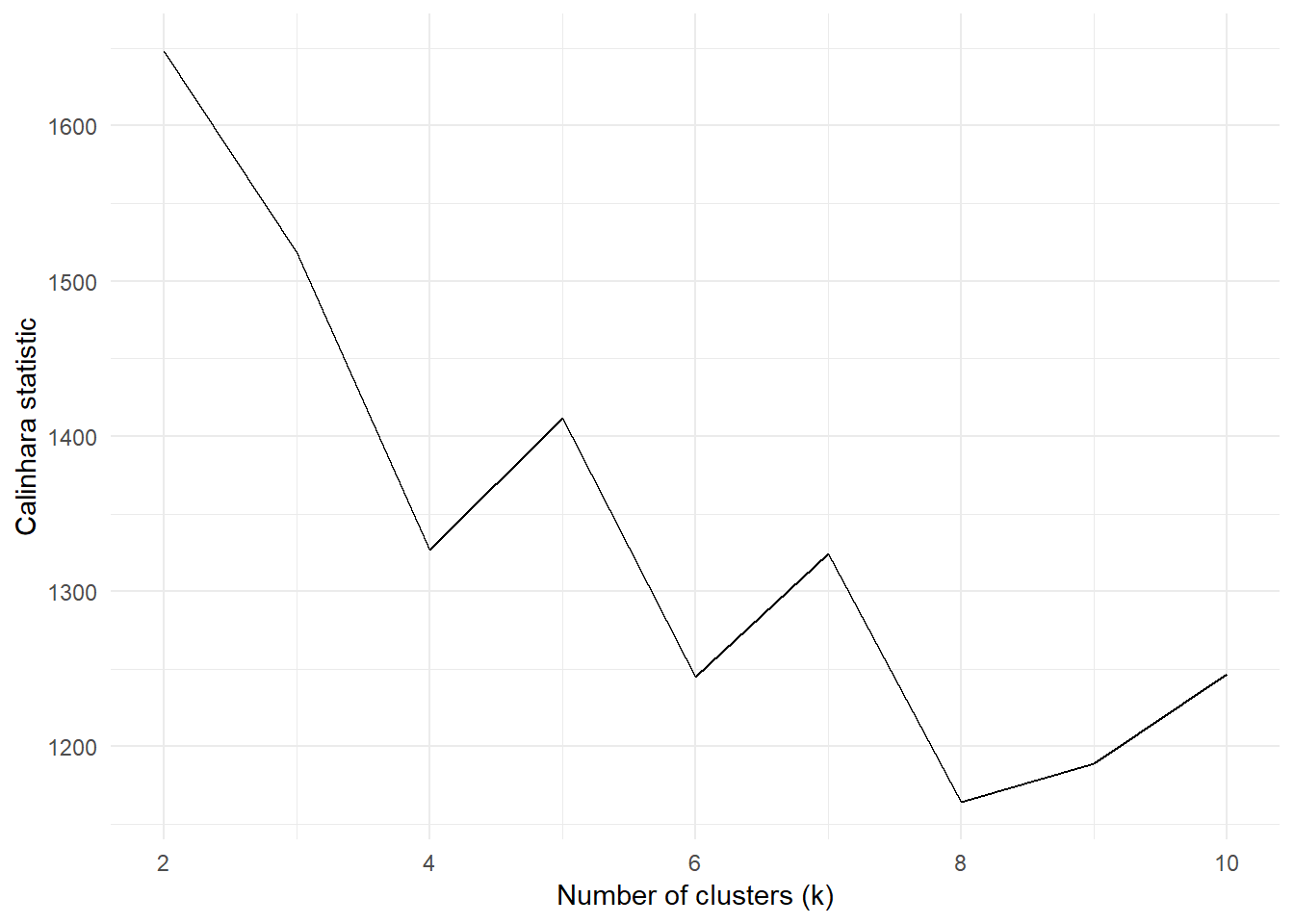From the above plot, the ideal k value to be selected is 2.

### Silhouette statistic

Silhouette statistic is the ratio of average distance within the cluster with average distance outside the cluster. If a(i) is the average distance between an observation i and other points in the cluster to which observation i belongs and b(i) is the minimum average distance between observation i and observations in other clusters. Then the Silhouette statistic is defined by

$S(i) = (\frac{b(i)-a(i)}{Max[a(i), b(i)]})$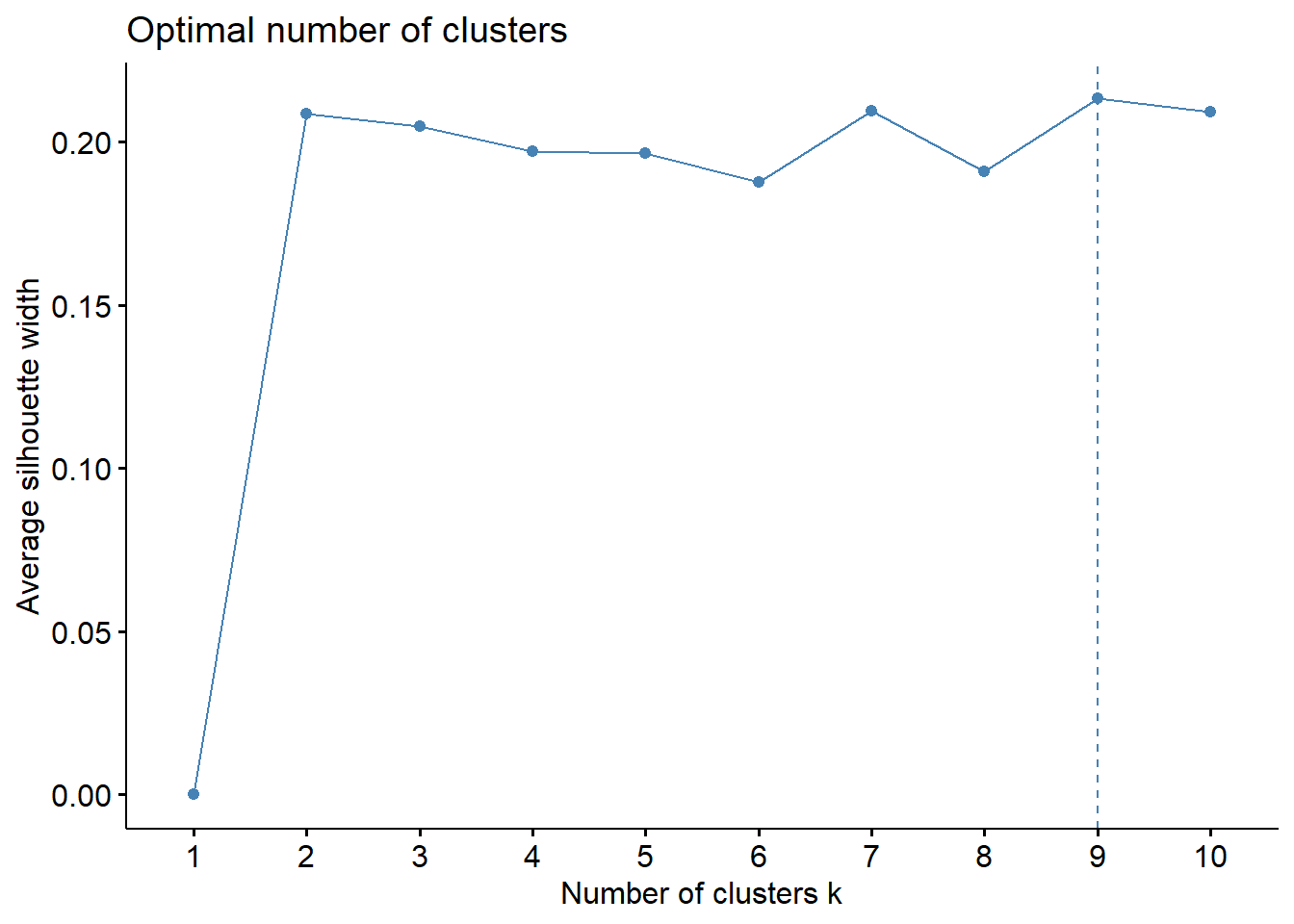The ideal k using silhouette 2 to 6 as there is not much reduction in silhouette metric.

## Elbow curve

Elbow curve is based on within-cluster variation. The location of a bend (knee) in the plot is generally considered as an indicator of the appropriate number of clusters. (see blog on hierarchical clustering for more)

fviz_nbclust(cluster_data, kmeans, method = "wss")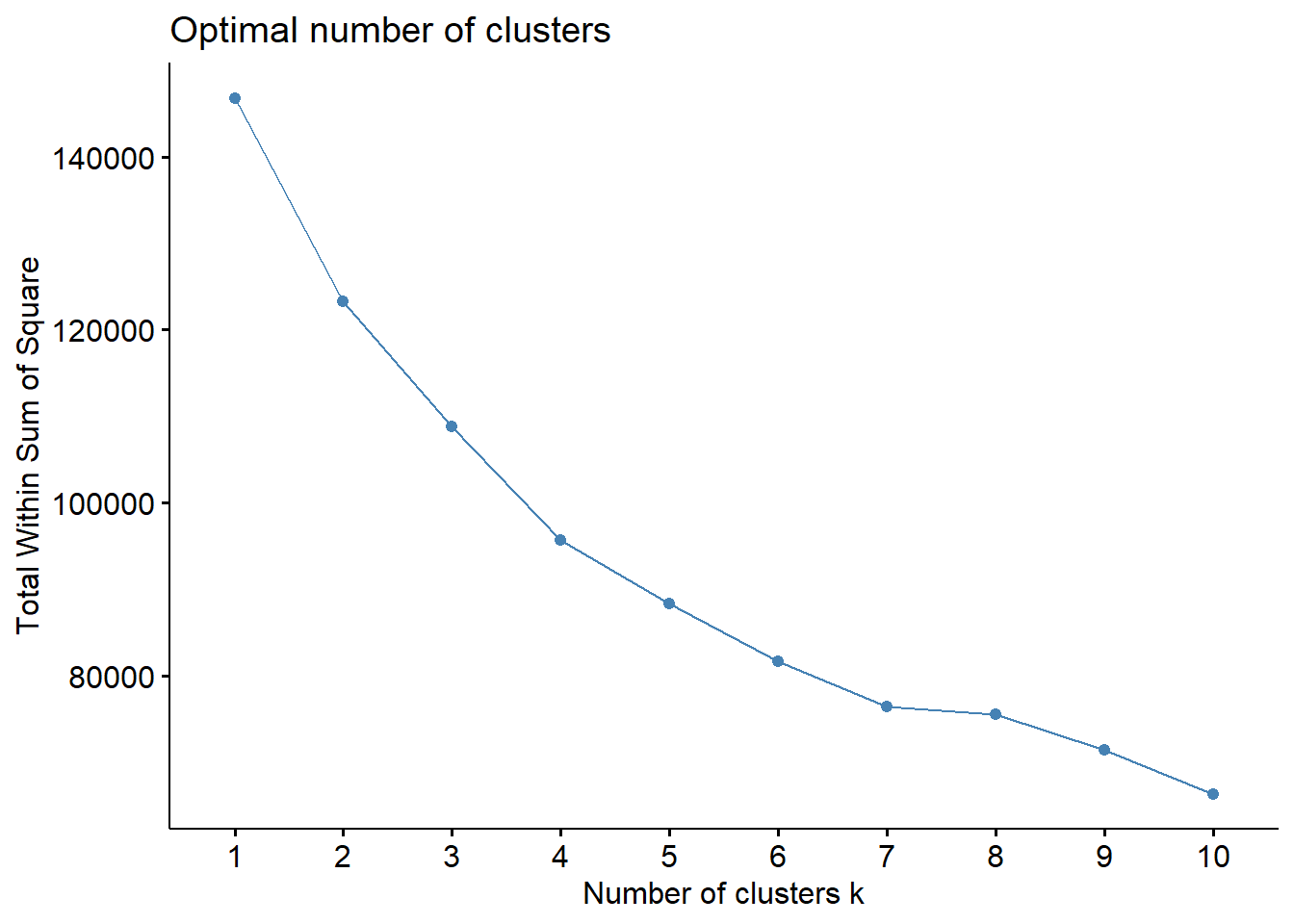From the above three metrics, the optimum value of k is 3.

## Analyzing the cluster

K-means can be computed in R with the kmeans function. We will group the data into three clusters (centers = 3). The kmeans function also has an nstart option that attempts multiple initial configurations and reports on the best one. For example, adding nstart = 25 will generate 25 initial configurations. This approach is often recommended.

final_cluster <- kmeans(cluster_data, centers = 3, nstart = 25)

The final clusters can be visualized below. In the below figure, all the columns are reduced to two columns for visualization using PCA.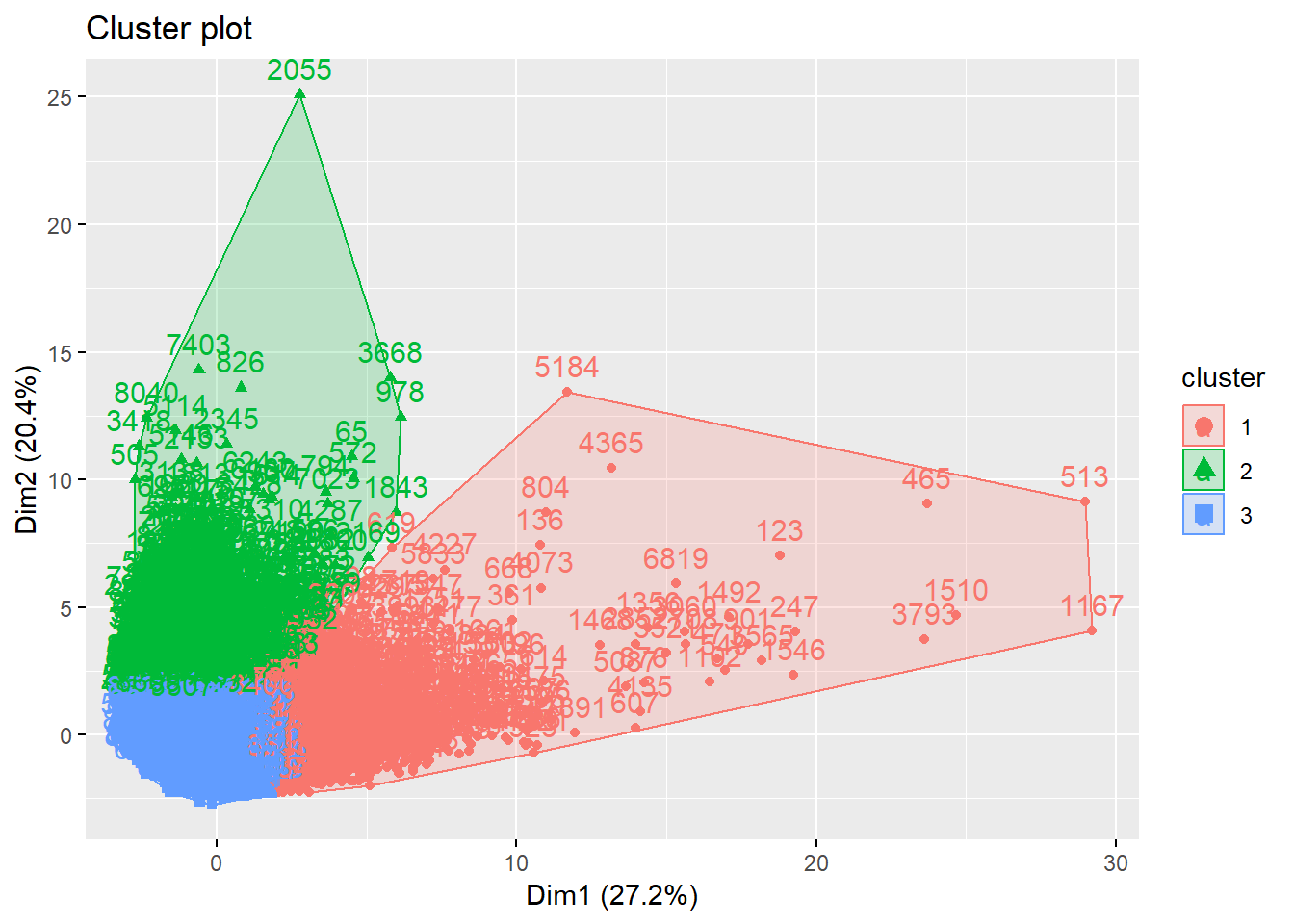In each column, the variation between the three clusters can be analysed using the following plots.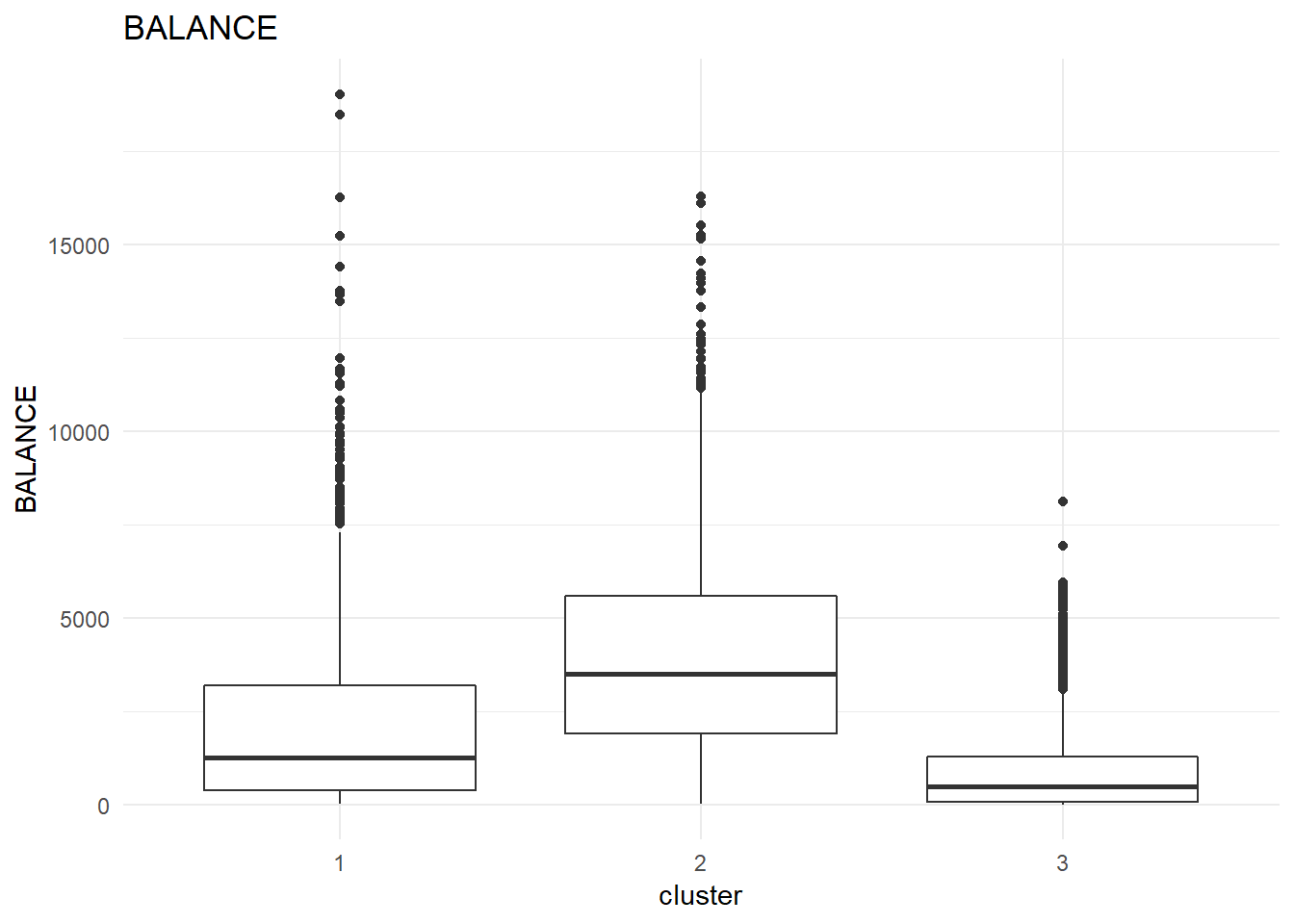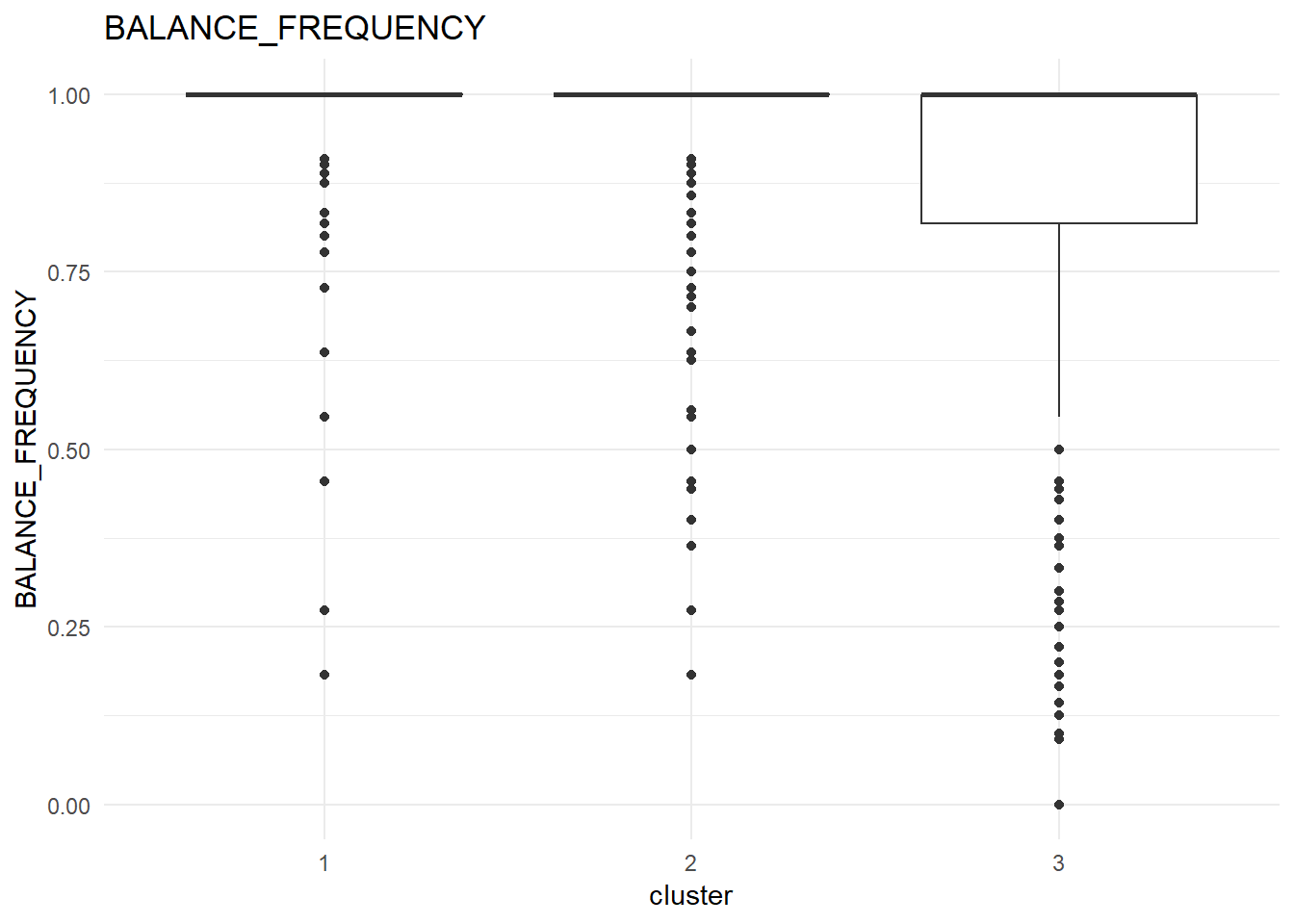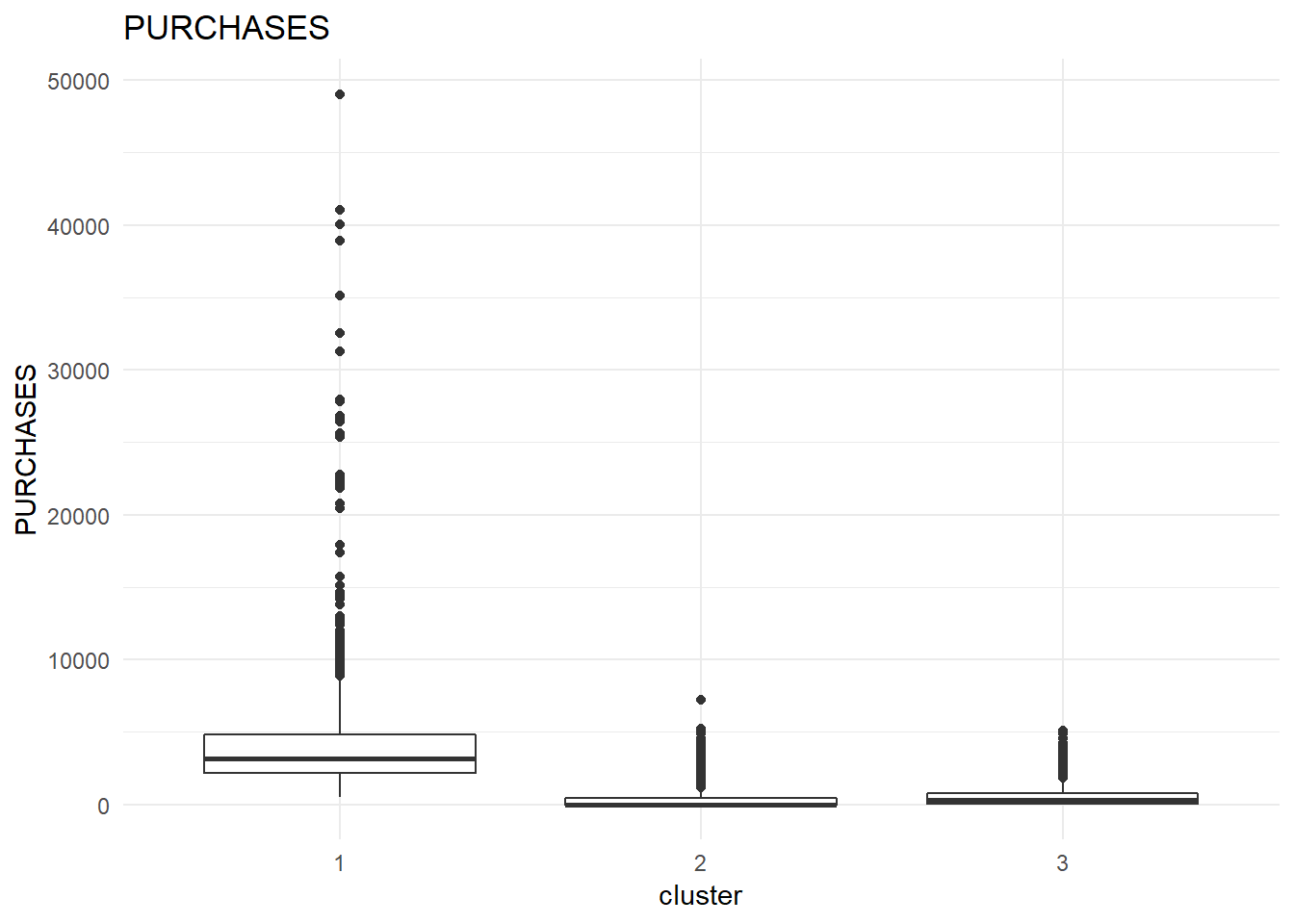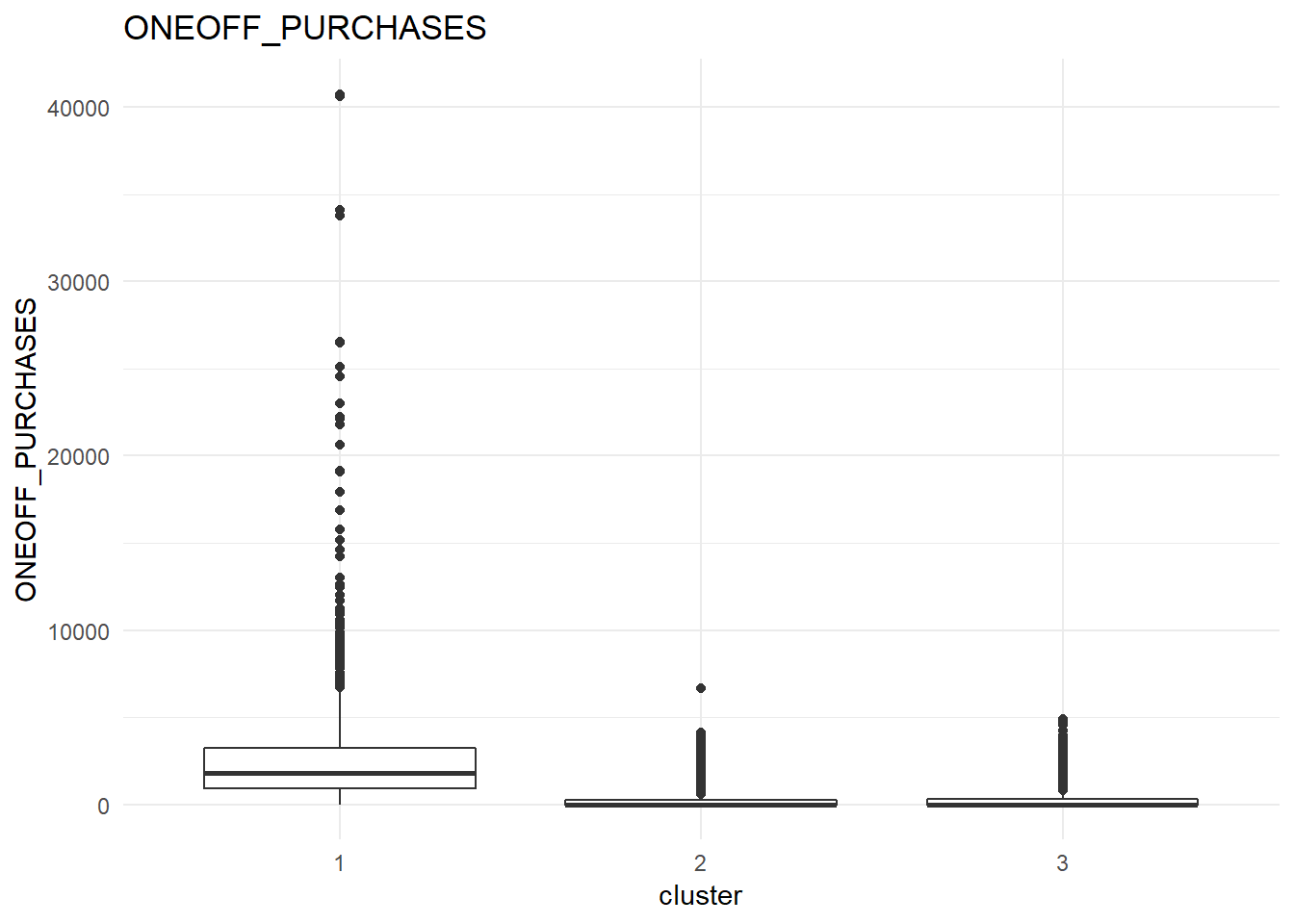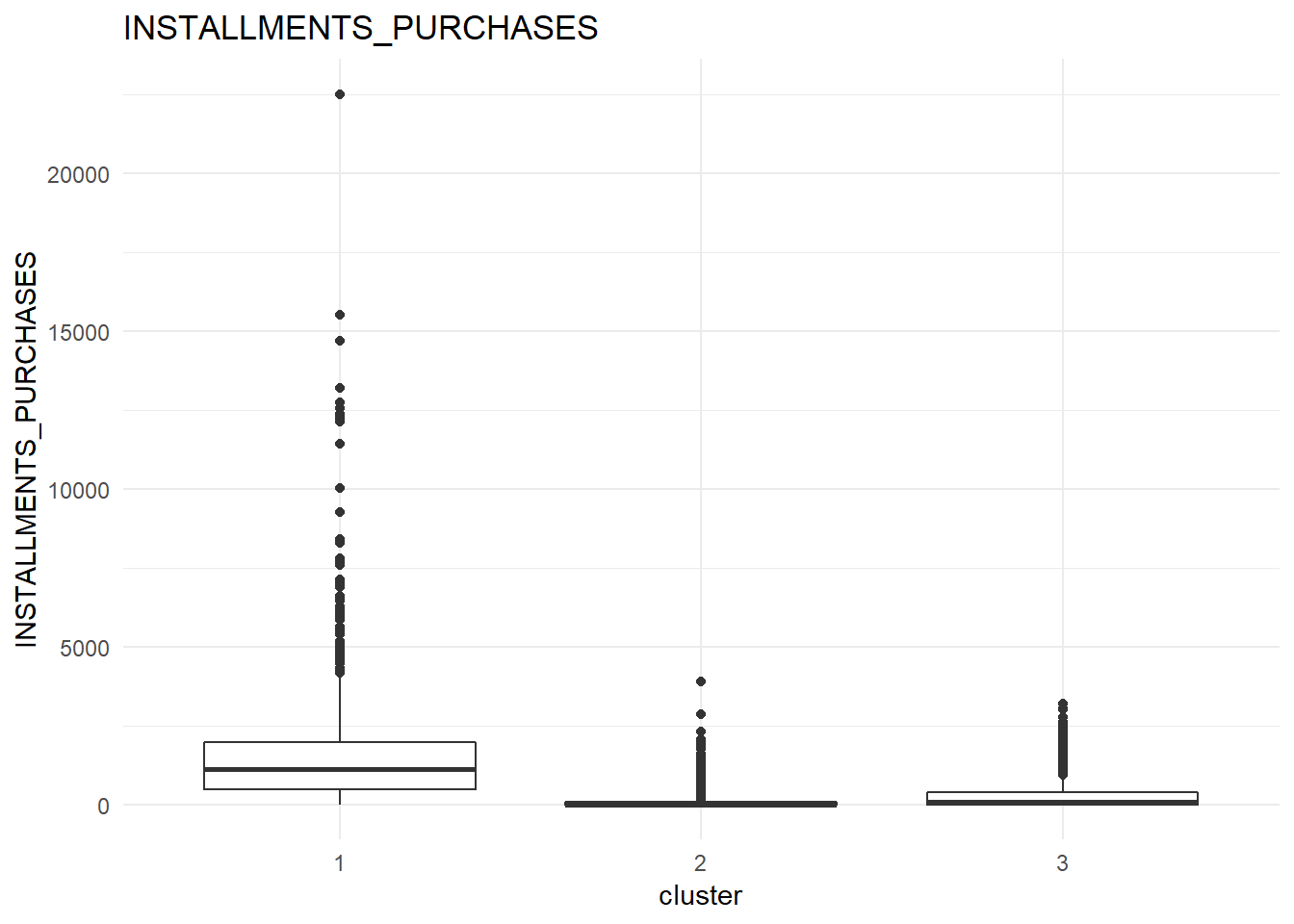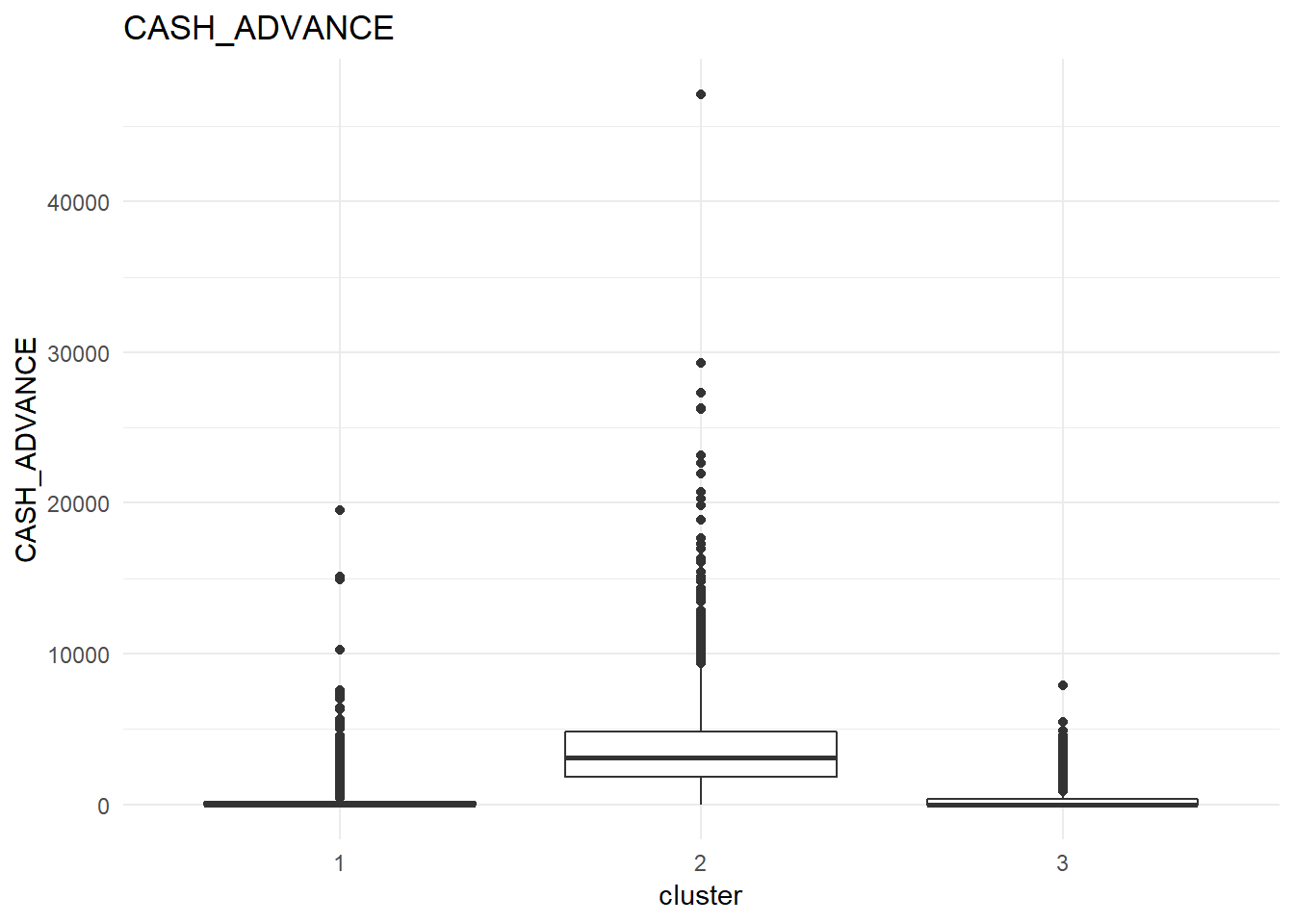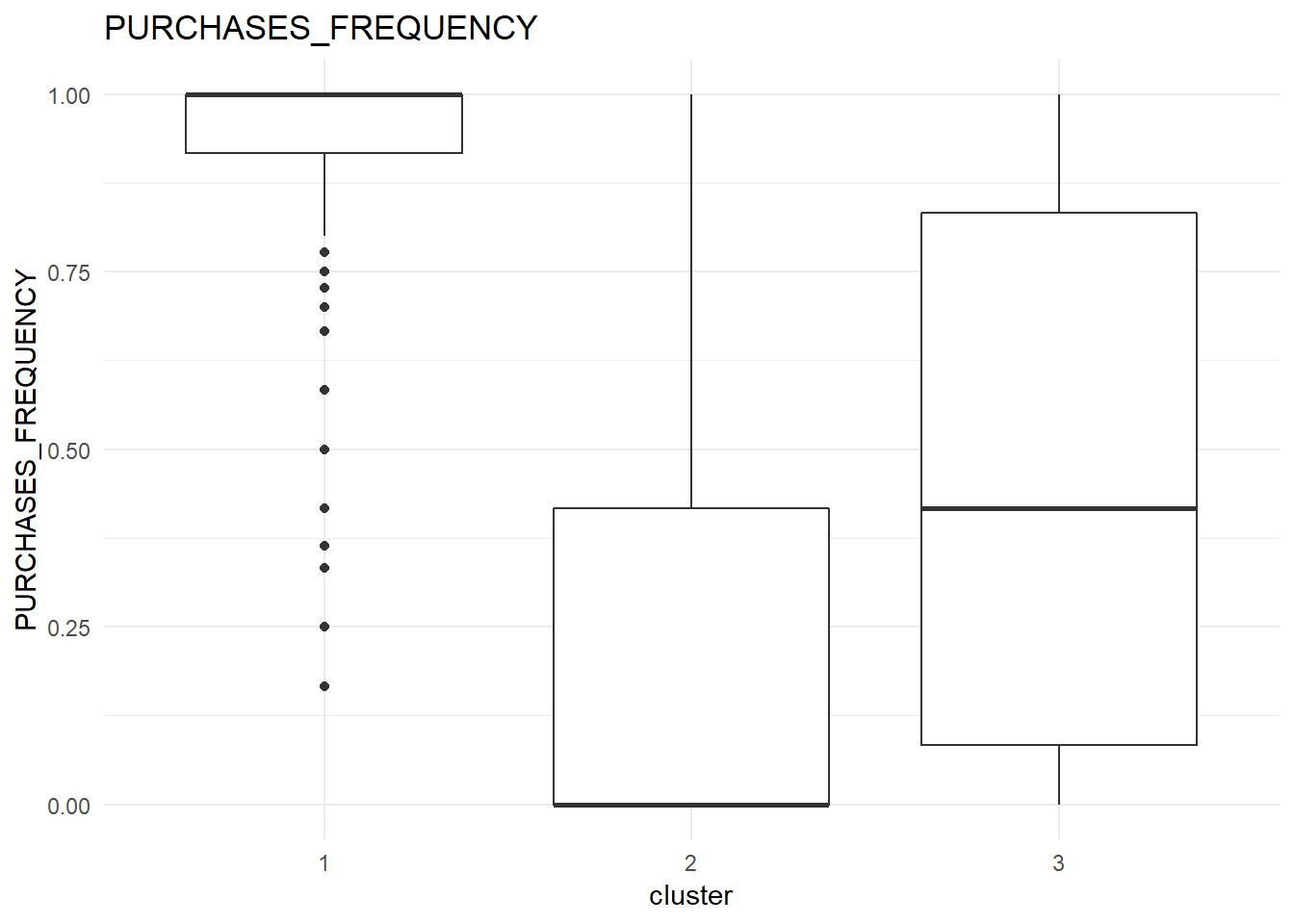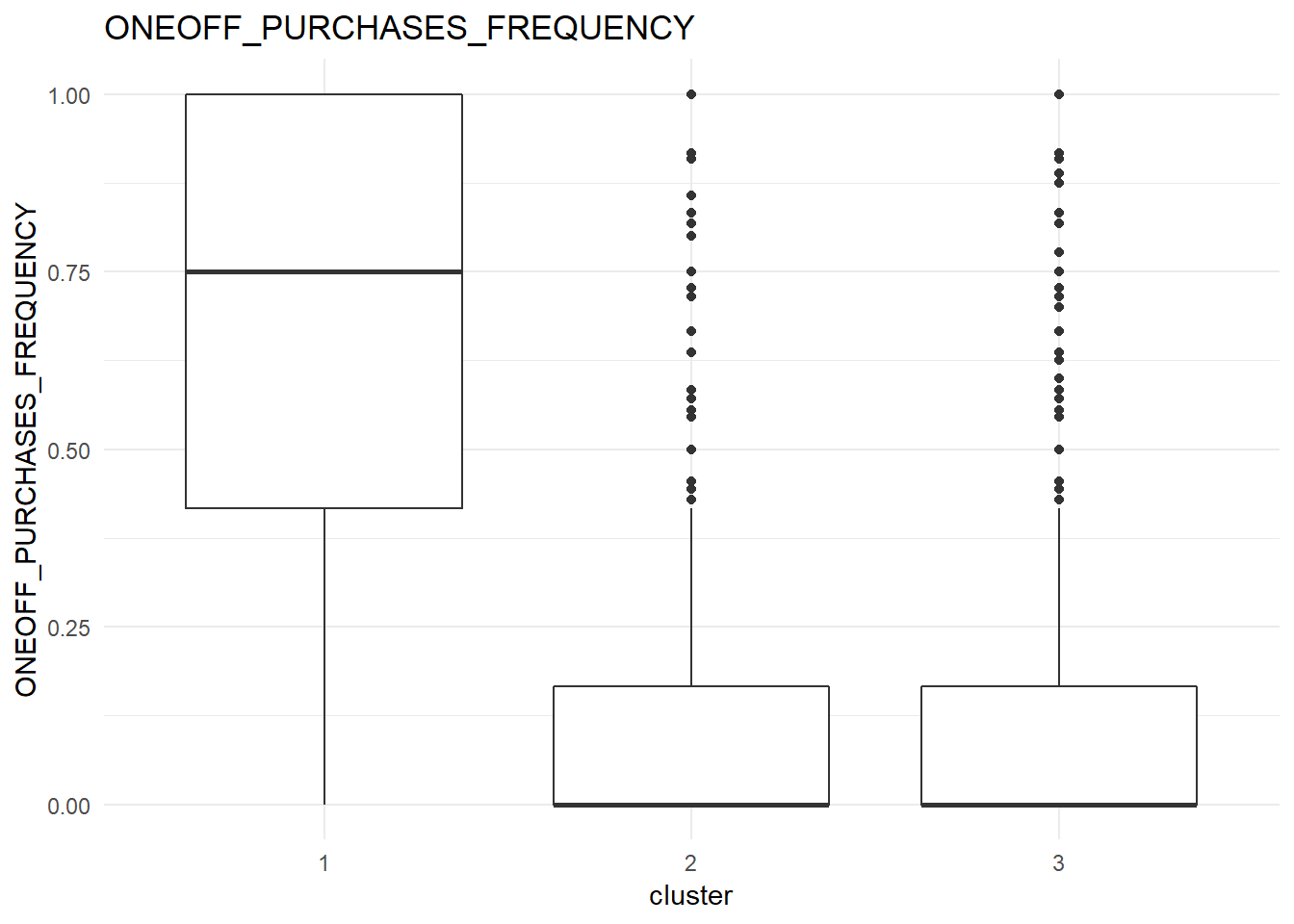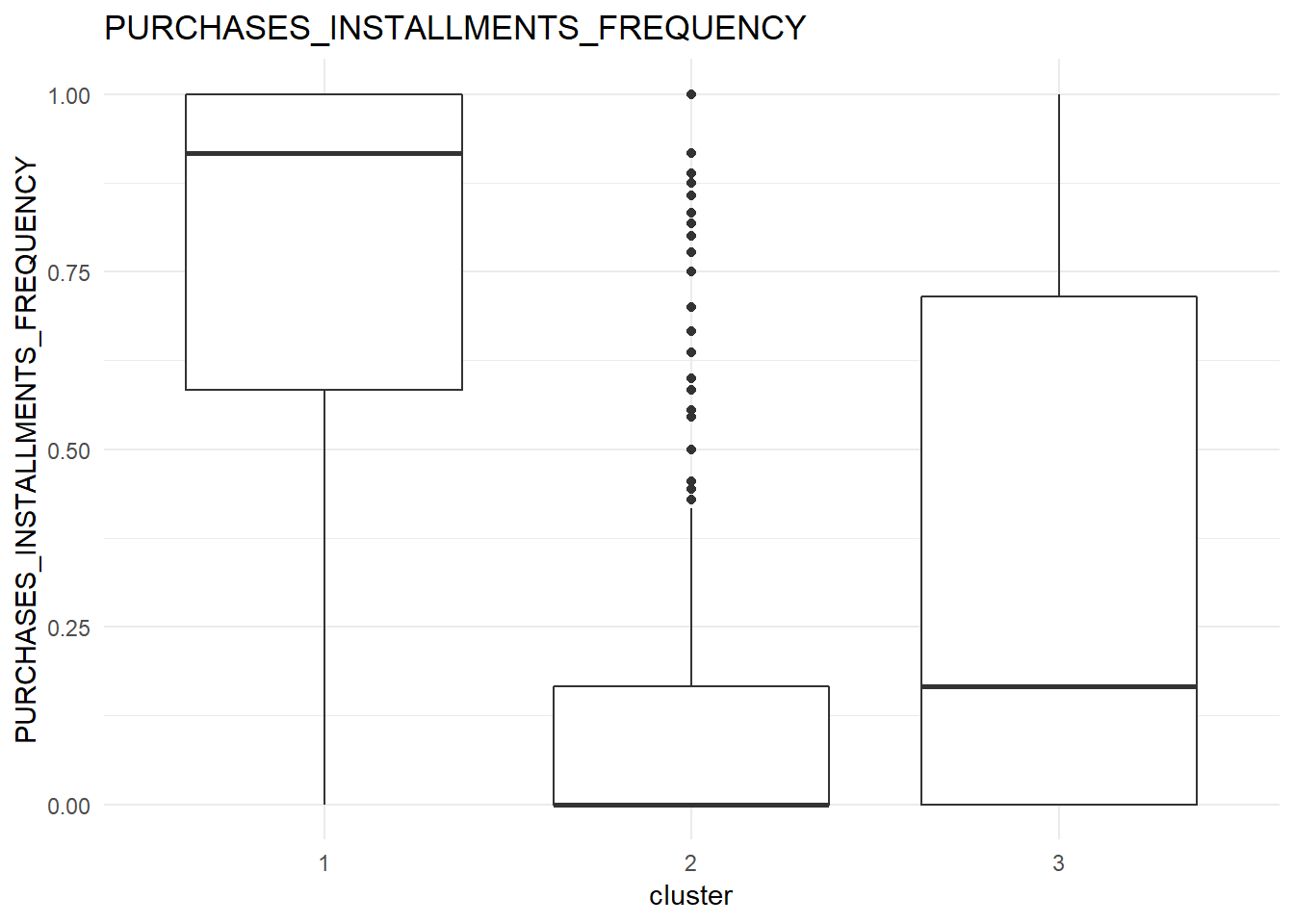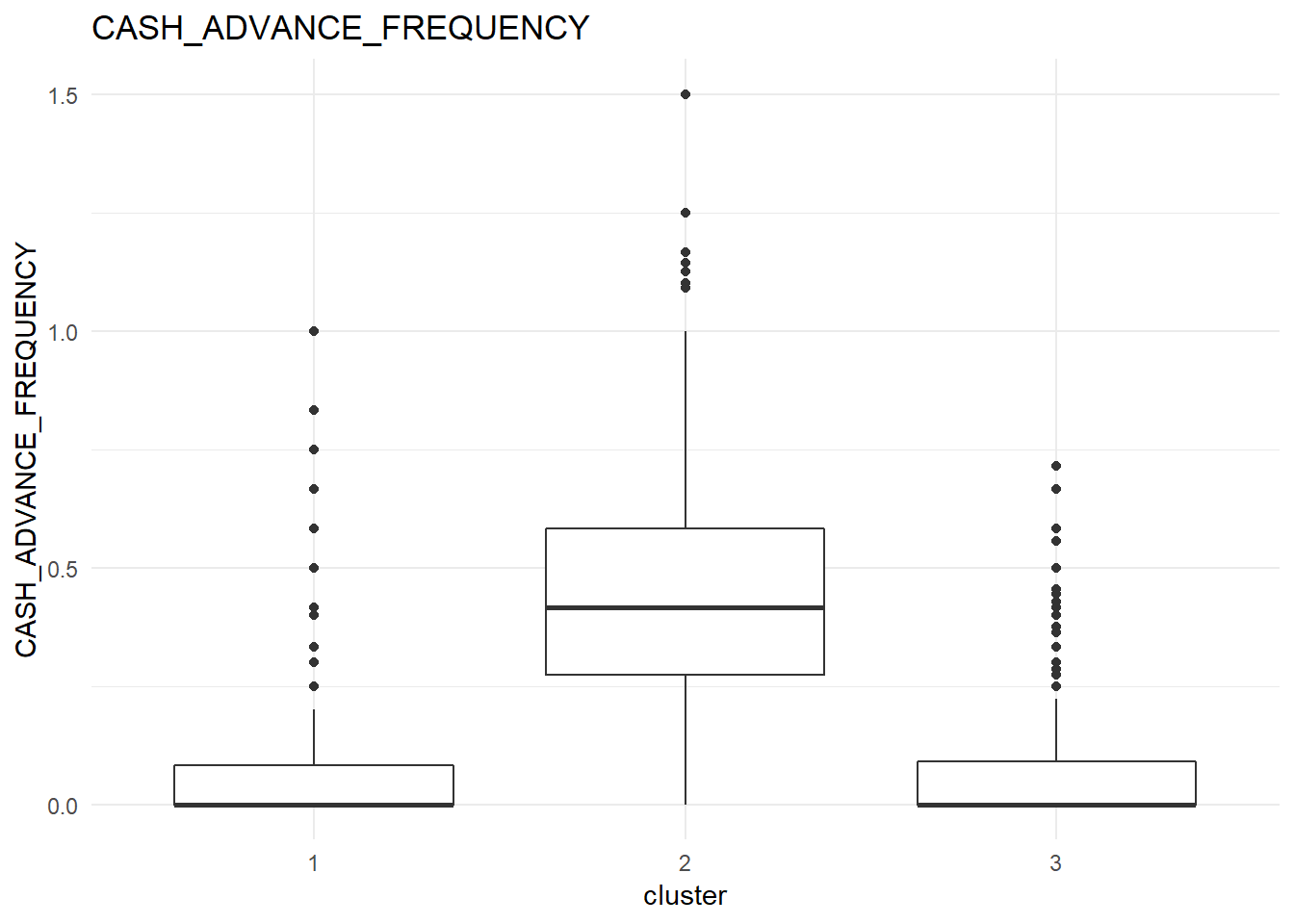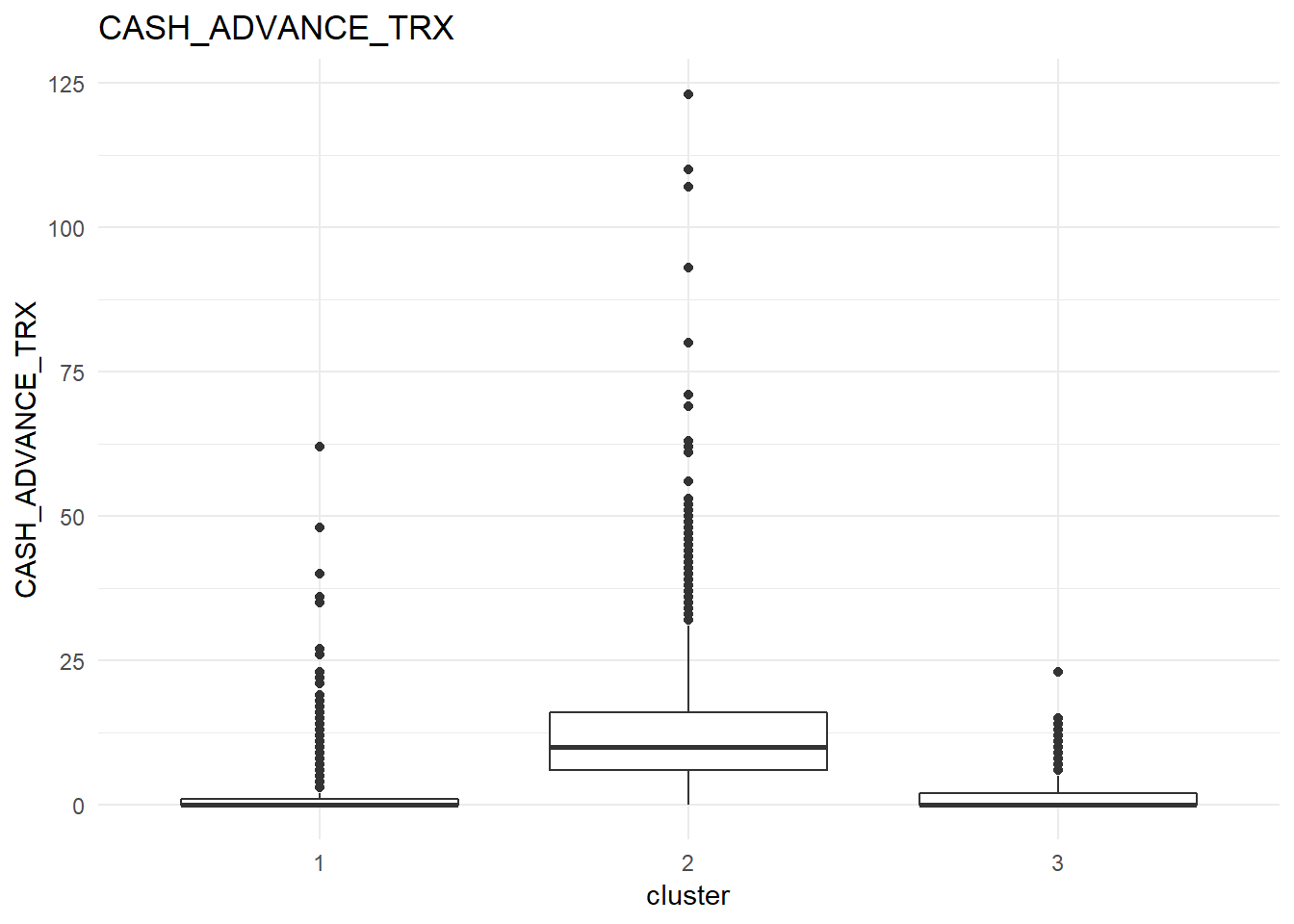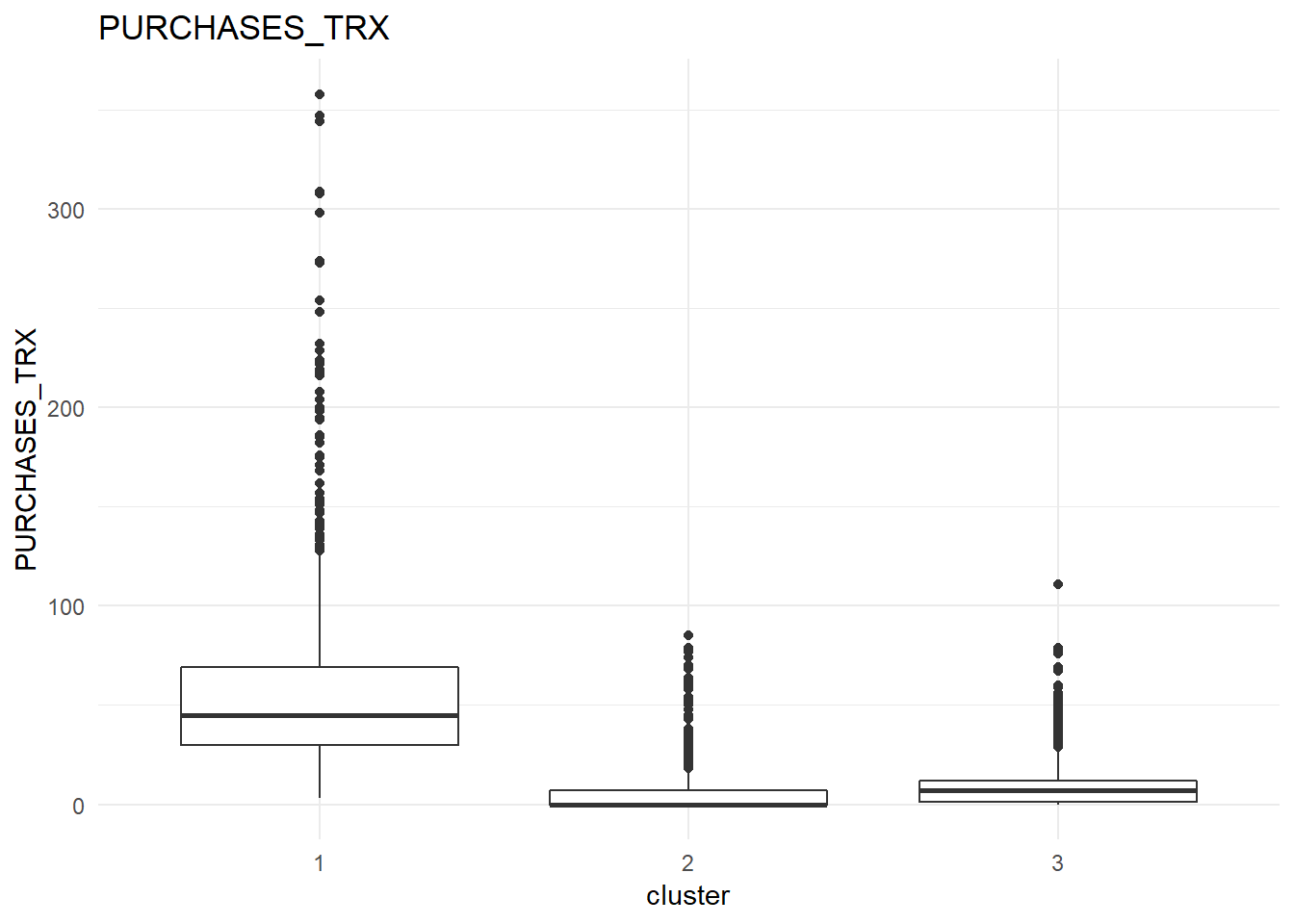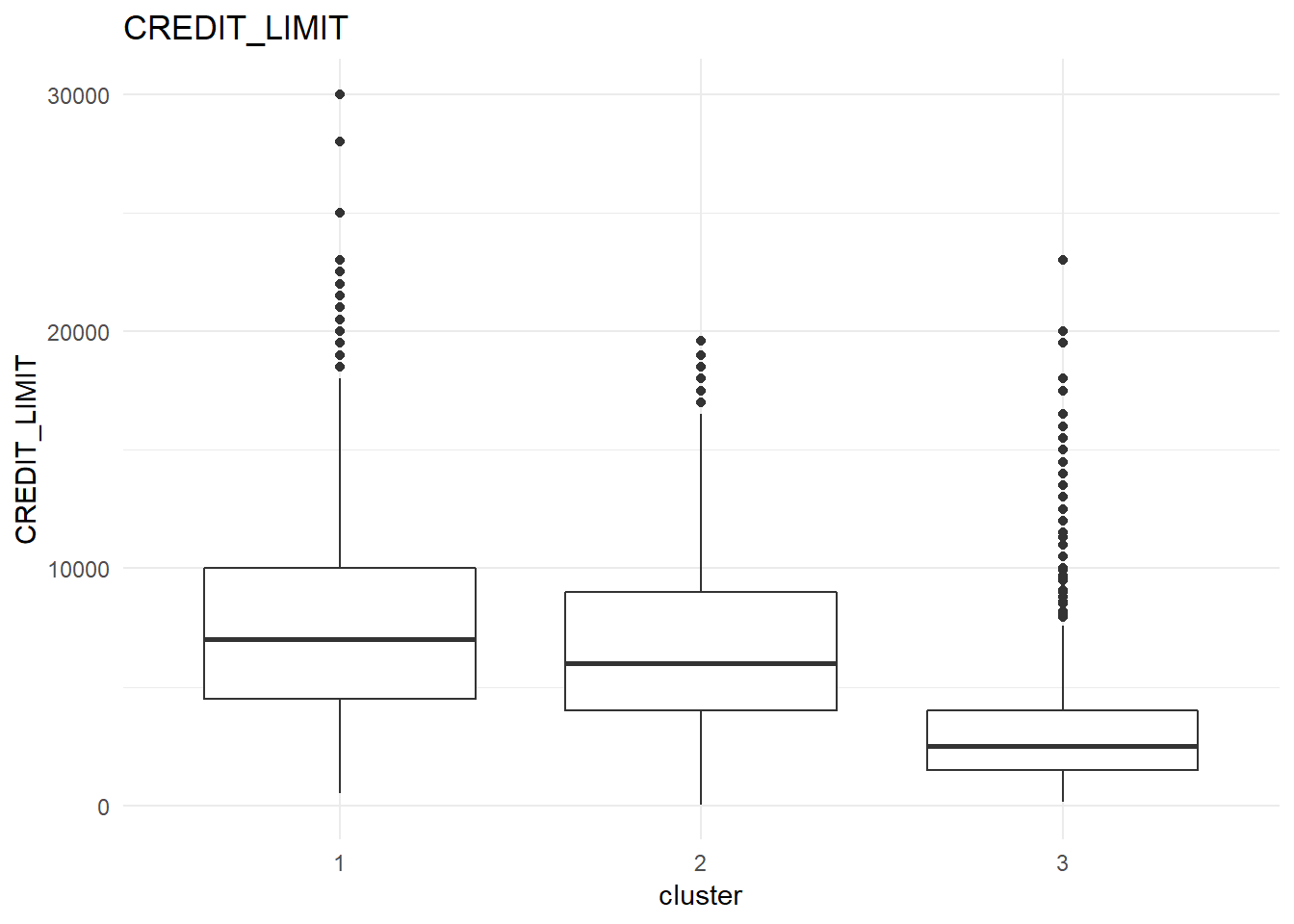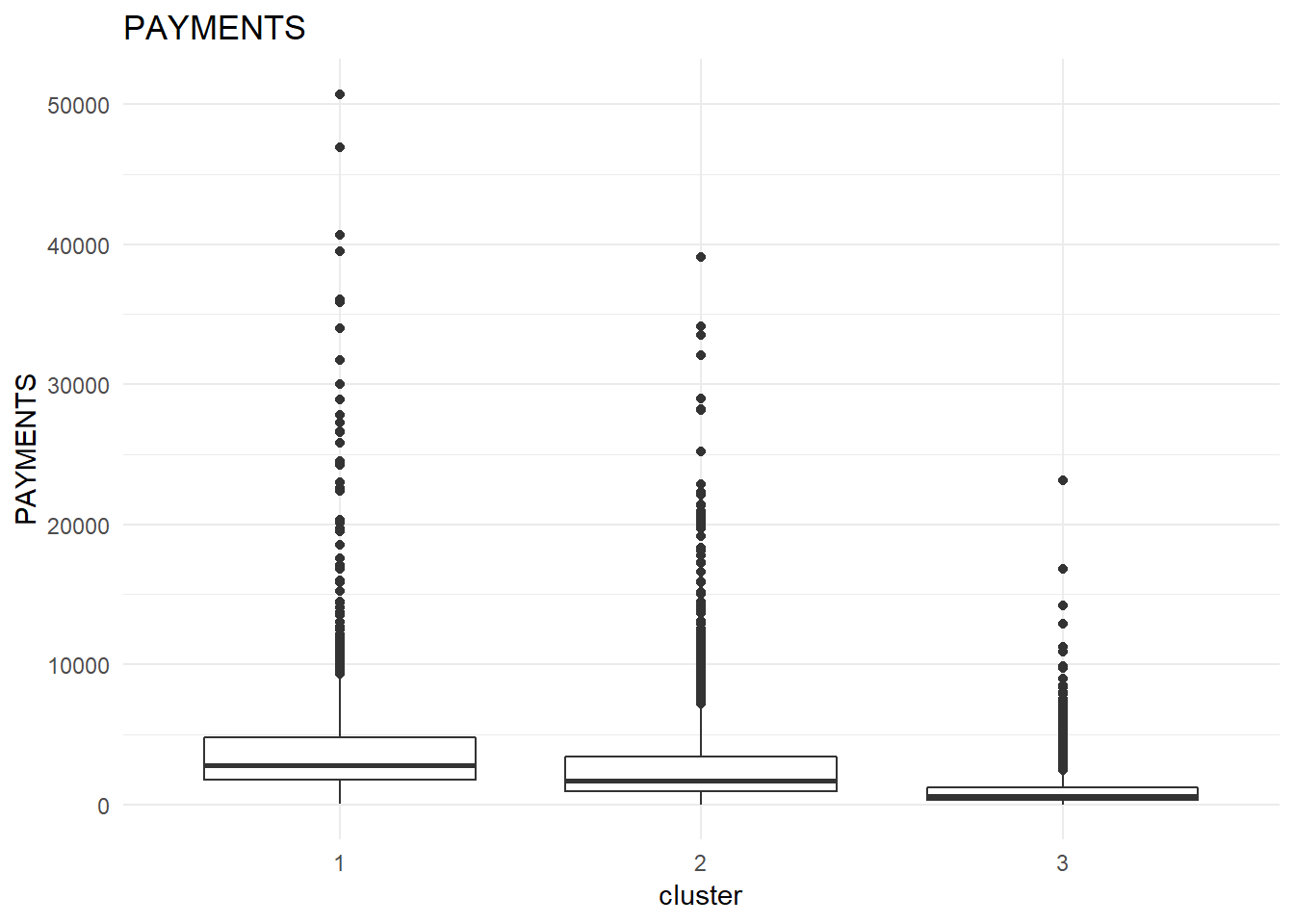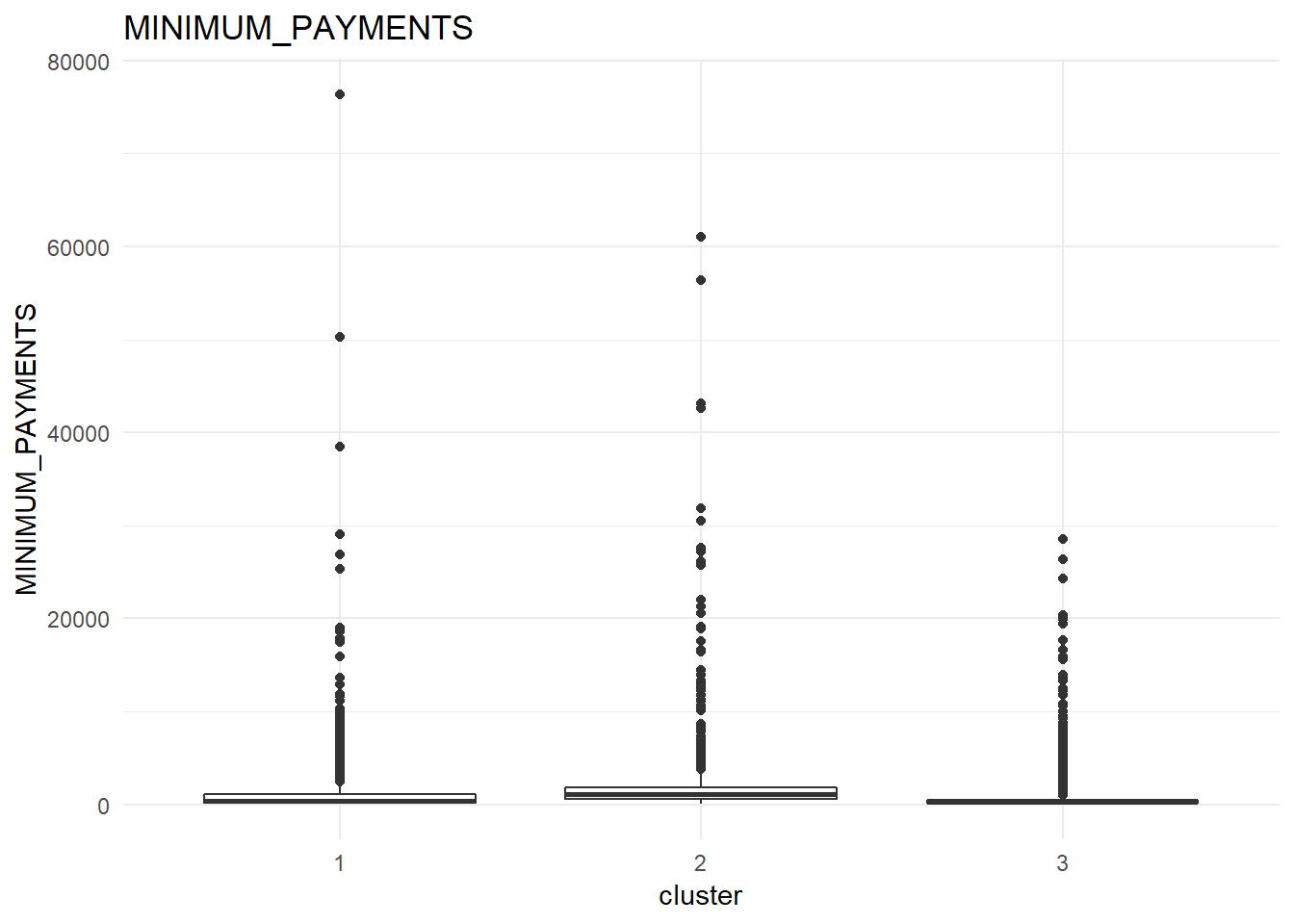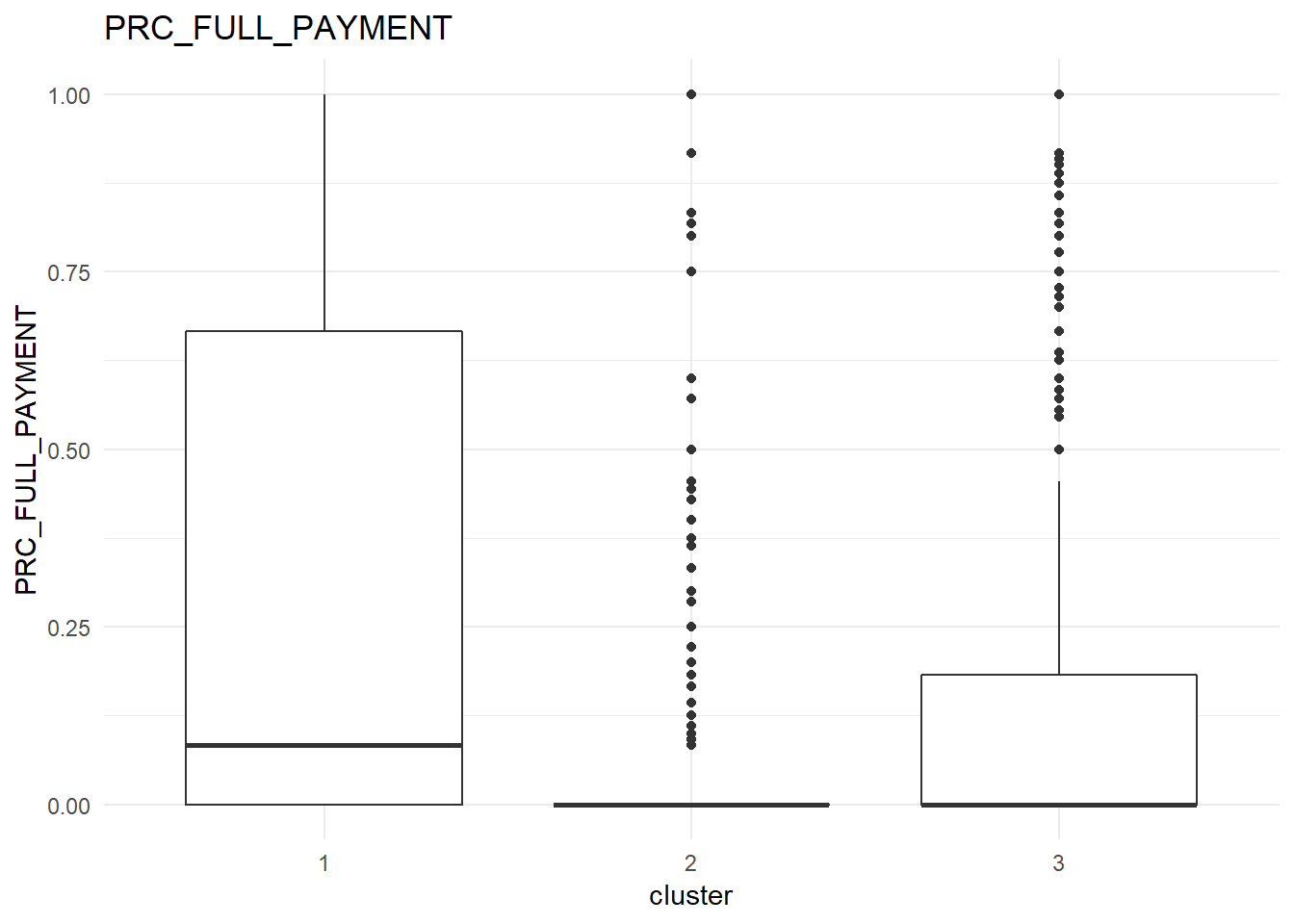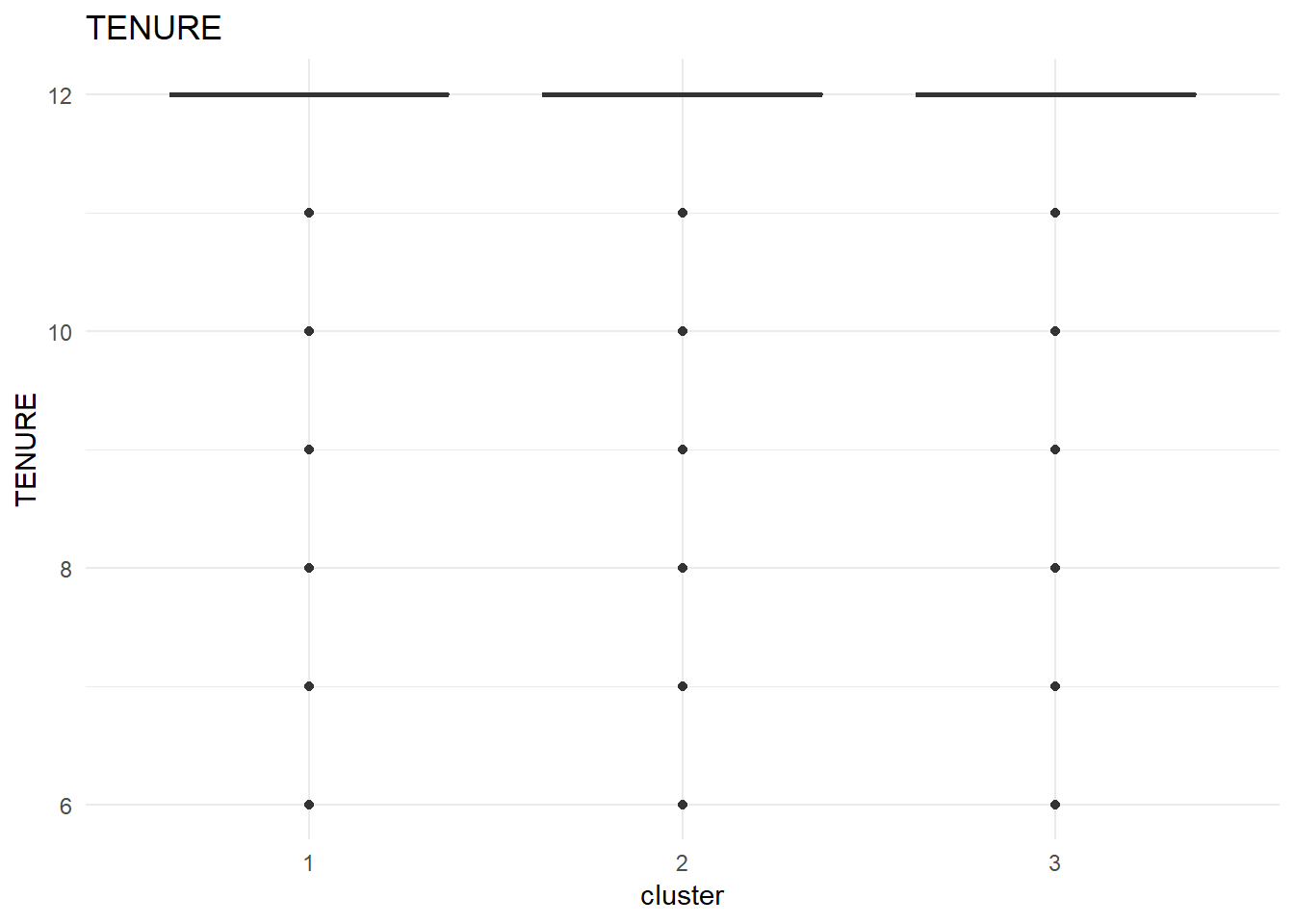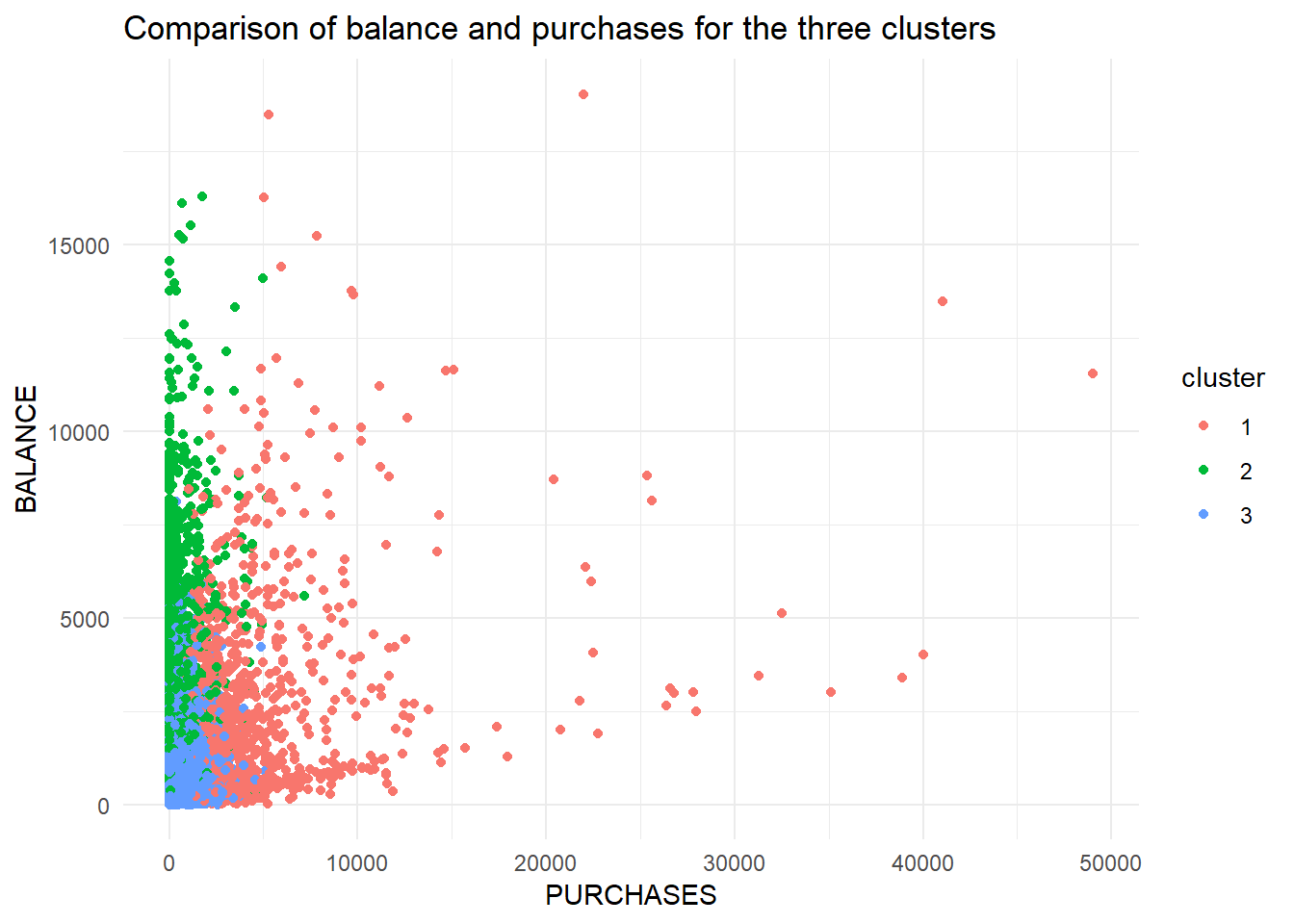From the comparison plot, we can observe the properties of the three clusters. They are:

##### Cluster 1

They are customers who purchase lesser amounts using the card but have reasonably high balance in their account.

##### Cluster 2

They are customers who have a low balance in their account and also purchase less using the account.

##### Cluster 3

They are customers who purchase in higher value using their card.
___________________________________________________